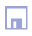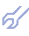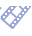This algorithm can be implemented simply in a such way: if you have Gauss procedure of system of linear equations solution, then you put it in a loop for generation of all possible combination of inputs and evaluate results on separate subsample (not used for learning) by error criterion. Then you need to select the best model among calculated only. The extended Combinatorial GMDH algorithm briefly can be described in such way: Input of parameters and data. Preliminary data handling: norming, generation of secondary non-linear variables... Selection of criterion: regularity, balance or cross-validation type and division of input data sample into learning and test subsample. For regularity criterion it's proposed to range all observations according to dispersion of function and take every third point to a test subsample. Three loops: for each function for each number of variables (at each layer)"" for all variables sets for given variables number^ selection of criterion; estimation of coefficients on learning sample and criterion value on test subsample. Coefficients are received by reducing of initial data array into quadratic array of normal equations, which are solved by LSE (Gauss or Choletsky) procedure; selection and saving of some best criterion values and corresponding ensemble of variables number for each layer; end of the third loop. At the end of the second loop: output of criterion value (as text or graph); termination of procedure on user demand; end of the second loop. At the end of the first loop: results output: regularization: selection the best models by secondary criterion among selected models; calculation of forecast value and errors; output of several best models. end of the first loop. End ^ - for more fast computation we propose to use the Garsaid counter for generation of variables ensembles [24,25]. "" - it was proposed for big number of variables to decrease time of full sorting procedure computing at 5-7 layer of sorting (or after some minutes): to range all variables according to criterion values; to select some most effective variables; continue sorting for selected variables (with addition or not of another variables). All questions about sorting procedure choice can be solved by comparison of values of external criterion.